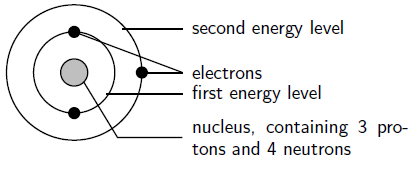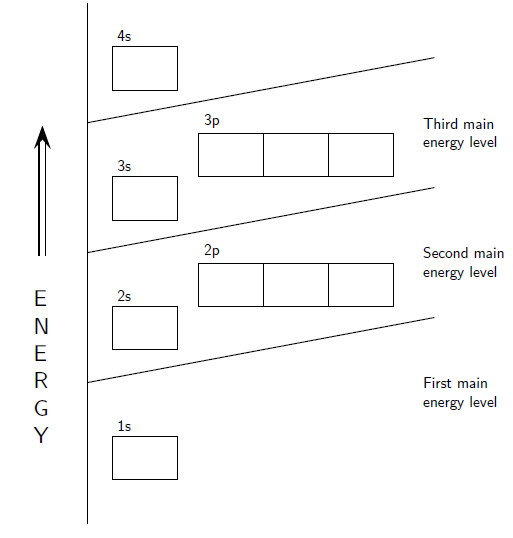## General Rules for Assigning Electrons to Atomic Orbitals

#### Learning Objective

• Determine the electron configuration for elements and ions, identifying the relation between electron shells and subshells.

#### Key Points

• If the energy of an atom is increased, an electron in the atom gets excited. To go back to its ground state, the electron releases energy. The energy of the light released when an electron drops in energy level is the same as the difference in energy between the two levels.
• Viewed simply, electrons are arranged in shells around an atom’s nucleus. Electrons closest to the nucleus will have the lowest energy. Electrons further away from the nucleus will have higher energy. An atom’s electron shell can accommodate 2n2 electrons (where n is the shell level).
• In a more realistic model, electrons move in atomic orbitals, or subshells. There are four different orbital shapes: s, p, d, and f. Within each shell, the s subshell is at a lower energy than the p. An orbital diagram is used to determine an atom’s electron configuration.
• There are guidelines for determining the electron configuration of an atom. An electron will move to the orbital with lowest energy. Each orbital can hold only one electron pair. Electrons will separate as much as possible within a shell.

#### Terms

• quantizationThe process of approximating a continuous signal by a set of discrete symbols or integer values.
• frequencyThe number of occurrences of a repeating event per unit of time.

## Energy of Electrons in Atomic Orbitals

The central structure of an atom is the nucleus, which contains protons and neutrons. This nucleus is surrounded by electrons. Although these electrons all have the same charge and the same mass, each electron in an atom has a different amount of energy. Electrons with the lowest energy are found closest to the nucleus, where the attractive force of the positively charged nucleus is the greatest. Electrons that have higher energy are found further away.

## Energy Quantization

When the energy of an atom is increased (for example, when a substance is heated), the energy of the electrons inside the atom is also increased—that is to say, the electrons get excited. For the excited electron to go back to its original energy, or ground state, it needs to release energy. One way an electron can release energy is by emitting light. Each element emits light at a specific frequency (or color) upon heating that corresponds to the energy of the electronic excitation.

It is helpful to think of this like going up a flight of steps. If you don’t lift your foot enough, you will bump into the step and be stuck on the ground level. You need to lift your foot to the height of the step to move on. The same goes for electrons and the amount of energy they can have. This separating of electrons into energy units is called quantization of energy because there are only certain quantities of energy that an electron can have in an atom. The energy of the light released when an electron drops down from a higher energy level to a lower energy level is the same as the difference in energy between the two levels.

## Electron Shells

We will start with a very simple way of showing the arrangement of electrons around an atom. Here, electrons are arranged in energy levels, or shells, around the nucleus of an atom. Electrons that are in the first energy level (energy level 1) are closest to the nucleus and will have the lowest energy. Electrons further away from the nucleus will have higher energy. An atom’s electron shell can accommodate 2n2 electrons, where n is the energy level. For example, the first shell can accommodate 2 x (1)2 or 2 electrons. The second shell can accommodate 2 x (2)2, or 8, electrons.The arrangement of electrons in a lithium atomLithium (Li) has an atomic number of 3, meaning that in a neutral atom, the number of electrons will be 3. The energy levels are shown as concentric circles around the central nucleus, and the electrons are placed from the inside out. The first two electrons are found in the first energy level, and the third electron is found in the second energy level.

As an example, fluorine (F), has an atomic number of 9, meaning that a neutral fluorine atom has 9 electrons. The first 2 electrons are found in the first energy level, and the other 7 are found in the second energy level.

## Atomic Orbitals

Though electrons can be represented simply as circling the nucleus in rings, in reality, electrons move along paths that are much more complicated. These paths are called atomic orbitals, or subshells. There are several different orbital shapes—s, p, d, and f—but we will be focusing mainly on s and p orbitals for now. The first energy level contains only one s orbital, the second energy level contains one s orbital and three p orbitals, and the third energy level contains one s orbital, three p orbitals, and five d orbitals. Within each energy level, the s orbital is at a lower energy than the p orbitals.Orbital diagramThe positions of the first ten orbits of an atom on an energy diagram. Note that each block is able to hold two electrons.

An orbital diagram helps to determine the electron configuration of an element. An element’s electron configuration is the arrangement of the electrons in the shells. There are a few guidelines for working out this configuration:

• Each orbital can hold only two electrons. Electrons that occur together in an orbital are called an electron pair.
• An electron will always try to enter the orbital with the lowest energy.
• An electron can occupy an orbital on its own, but it would rather occupy a lower-energy orbital with another electron before occupying a higher-energy orbital. In other words, within one energy level, electrons will fill an s orbital before starting to fill p orbitals.
• The s subshell can hold 2 electrons.
• The p subshells can hold 6 electrons.

Electron configurations can be used to rationalize chemical properties in both inorganic and organic chemistry. It is also used to interpret atomic spectra, the method used to measure the energy of light emitted from elements and compounds.